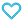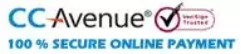# The Elements of Coordinate Geometry Part-1

140.00

The Elements of Coordinate Geometry Part-1 book is useful to learn fundamentals of coordinate geometry. It contains approximate 1100 examples. The content has presented in the manner suitable for beginners and junior students. Answers for unsolved problems are given at the end of the book.

912 in stock

Qty:Add to WishlistTable of Content:
• 1. Introduction
• 2. Coordinates, Lengths of Straight Lines and Areas of
• Triangles
•  Polar coordinates
• 3. Locus, Equation to a Locus
• 4. The Straight Line, Rectangular Coordinates
•  Straight line through two points
•  Angle between two given straight lines
•  Conditions that they may be parallel and perpendicular
•  Length of a perpendicular
•  Bisectors of angles
• 5. The Straight Line (Continued)
• Polar Equations and Oblique Coordinates
•  Equations involving an arbitrary constant
•  Examples of loci
• 6. On Equations Representing Two or More Straight Lines
•  Angle between two lines given by one equation
•  General equation of the second degree
• 7. Transformation of Coordinates
•  Invariants
• 8. The Circle
•  Equation to a tangent
•  Pole and polar
•  Equation to a circle in polar coordinates
•  Equation referred to oblique axes
•  Equations in terms of one variable
• 9. Systems of Circles
•  Orthogonal circles
•  Coaxal circles
• 10. The Parabola
•  Equation to a tangent
•  Some properties of the parabola
•  Pole and polar
•  Diameters
•  Equations in terms of one variable
• 11. The Parabola (Continued)
•  Loci connected with the parabola
•  Three normals passing through a given point
•  Parabola referred to two tangents as axes
• 12. The Ellipse
•  Auxiliary circle and eccentric angle
•  Equation to a tangent
•  Some properties of the ellipse
•  Pole and polar
•  Conjugate diameters
•  Four normals through any point
•  Examples of loci
• 13. The Hyperbola
•  Asymptotes
•  Equation referred to the asymptotes as axes
•  One variable. Examples
• 14. Polar Equation of a Conic Section, Its Focus being the Pole
•  Polar equation to a tangent, polar, and normal
• 15. General Equation of the Second Degree, Tracing of Curves
•  Particular cases of conic sections
•  Transformation of equation to centre as origin
•  Equation to asymptotes
•  Tracing a parabola
•  Tracing a central conic
•  Eccentricity and foci of general conic
• 16. The General Conic
•  Tangent
•  Conjugate diameters
•  Conics through the intersections of two conics
•  The equation S = uv
•  General equation to the pair of tangents drawn from
• any point
•  The director circle
•  The foci
•  The axes
•  Lengths of straight lines drawn in given directions
• to meet the conic.
•  Conics passing through four points
•  Conics touching four lines
•  The conic LM = R2
• 17. Miscellaneous Propositions
•  On the four normals from any point to a central conic
•  Confocal conics
•  Circles of curvature and contact of the third order
•  Envelopes